# What are

What are the four terms between 4 and 19?

a2 =  7
a3 =  10
a4 =  13
a5 =  16

### Step-by-step explanation:

${a}_{3}={a}_{2}+d=7+3=10$
${a}_{4}={a}_{3}+d=10+3=13$
${a}_{5}={a}_{4}+d=13+3=16$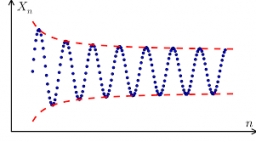Did you find an error or inaccuracy? Feel free to write us. Thank you!## Related math problems and questions:

• AP members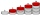What is the value of x2, x3, x4, x5…of the terms (of arithmetic progression) when x1 = 8 and x6 = 20?
• Difference AP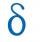Calculate the difference of arithmetic progression if the sum of its first 19 members Sn = 8075 and the first member is a1 = 20
• Geometric progressiobIf the sum of four consective terms of geometric progression is 80 and arithmetic mean of second and fourth term is 30 then find terms?
• Find the 19Find the 1st term of the GP ___, -6, 18, -54.
• Negative differenceFind four arithmetic progression members between 7 and -6.
• Arithmetic progression 2The 3rd term of an Arithmetic progression is ten more than the first term, while the fifth term is 15 more than the second term. Find the sum of the 8th and 15th terms of the Arithmetic progression if the 7th term is seven times the first term.
• GP - three membersThe second and third of a geometric progression are 24 and 12(c+1), respectively, given that the sum of the first three terms of progression is 76. determine the value of c.
• Sum of GP members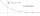Determine the sum of the GP 30, 6, 1.2, to 5 terms. What is the sum of all terms (to infinity)?
• Common difference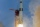The 4th term of an arithmetic progression is 6. If the sum of the 8th and 9th terms is -72, find the common difference.
• What are 2What are the two more terms of the GP a, ax, ax2, ax3, __, __?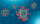A Street has 13 houses in a row. Some resident sin the first house tested positive for Covid-19. The virus spreads in 2 ways: It can spread to the next house or jump directly to the third house. Residents of house 2 can get infected in only one way, houseFind the sum of the geometric progression 3, 15, 75,… to six terms.The sum of the 17 different natural numbers is 154. Determine the sum of the two largest ones.Insert five arithmetic progression members between -7 and 3/2.The field has a shape of a rectangle having a length of 119 m and a width of 19 m. , How many meters have to shorten its length and increase its width to maintain its area and circumference increased by 24 m?Find the first six terms of the sequence. a1 = 7, an = an-1 + 6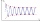The sum of four consecutive natural numbers is 114. Find them.